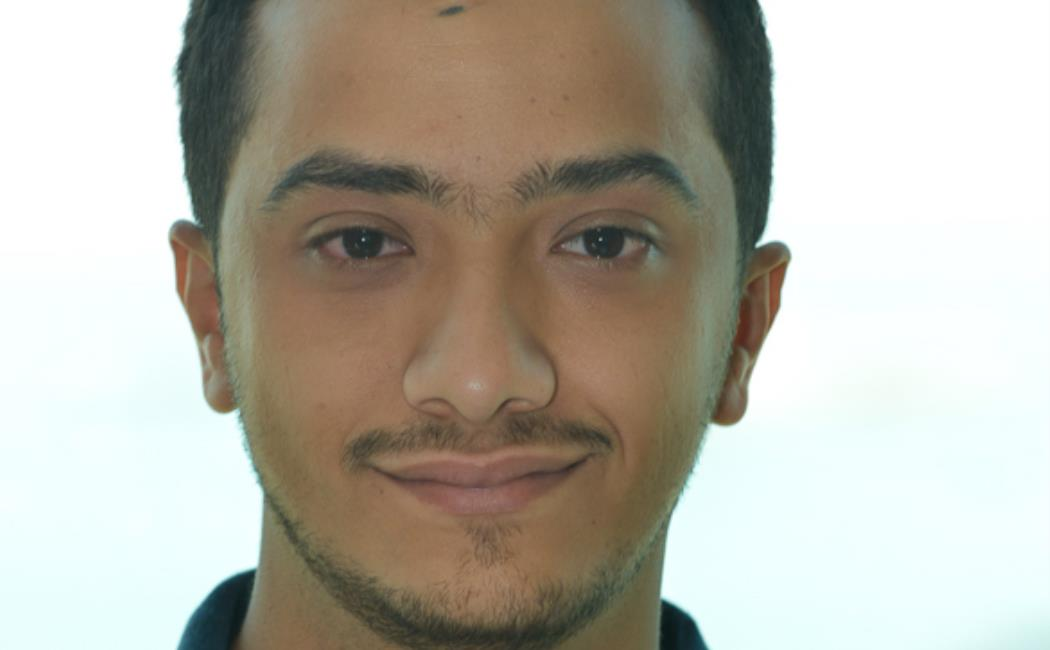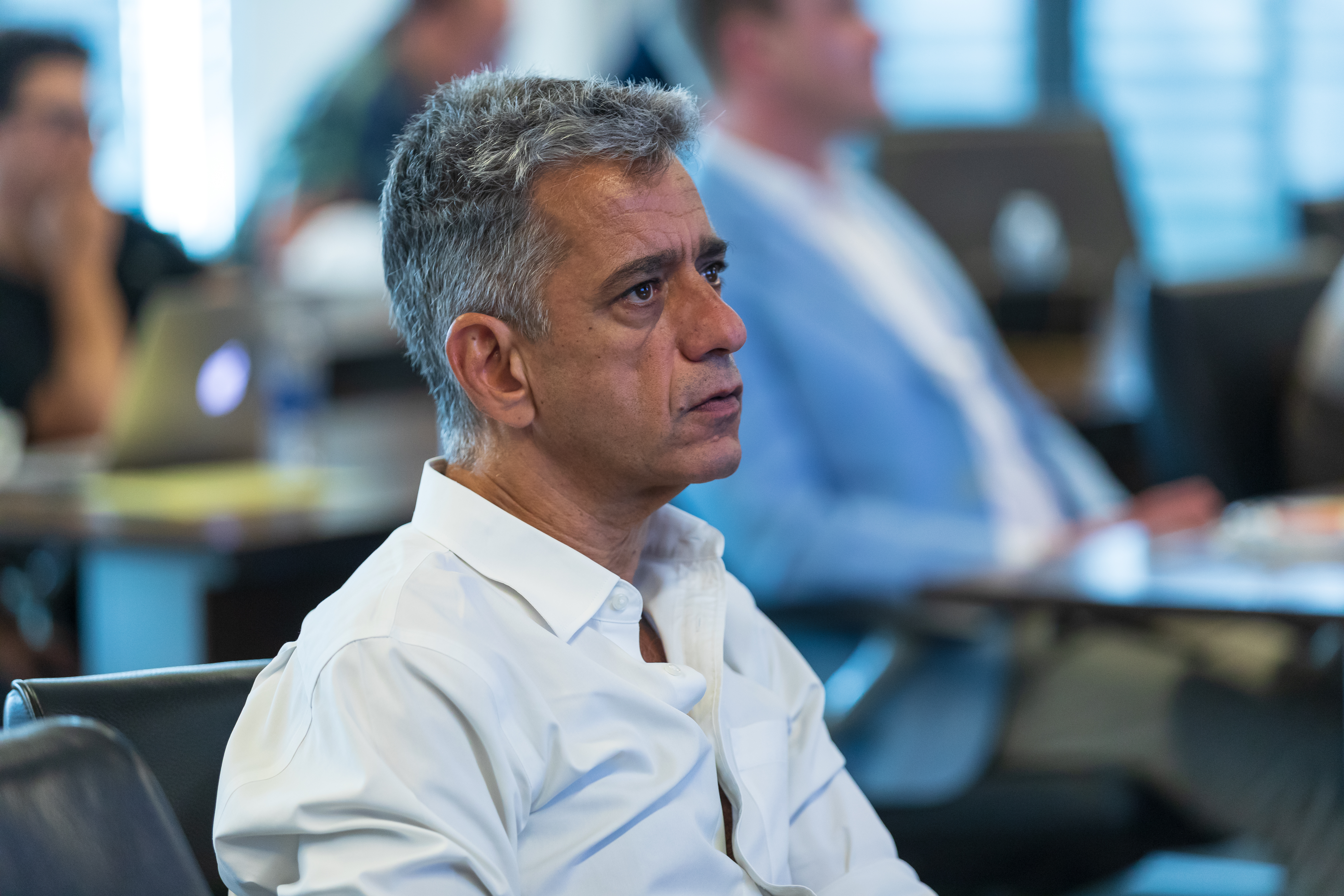## Zaid Sawlan successfully defended his PhD thesis

26 September, 2018

On September 26th, 2018, Zaid Sawlan successfully defended his PhD thesis entitled "Statistical analysis and Bayesian methods for fatigue life prediction and inverse problems in linear time dependent PDEs with uncertainties"
Committee Members:
Prof. Marco Scavino, UdelaR, Montevideo, Uruguay
Prof. Serge Prudhomme, Poly Montreal, Canada
Prof. Olivier LeMaitre, LIMSI, Paris, France
Prof. Haavard Rue, KAUST
Prof. Slim Alouini, KAUST
Prof. Fabio Nobile, EPFL, Lausanne, Switzerland External Examiner
Prof. Raul Tempone (KAUST, CEMSE, AMCS) Thesis Advisor

Abstract:

This work employs statistical and Bayesian techniques to analyze mathematical forward models with several sources of uncertainty. The forward models usually arise from phenomenological and physical phenomena and are expressed through regression-based models or partial differential equations (PDEs) associated with uncertain parameters and input data. One of the critical challenges in real-world applications is, for any proposed model, to estimate and quantify uncertainties of its unknown parameters using the available observations. To this purpose, methods based on the likelihood function, and Bayesian techniques constitute the two main statistical inferential approaches considered here, the latter providing a full probability distribution for the parameters of interest, named the posterior distribution. In the classical approach, bootstrap confidence intervals procedures are used to assess the variability of the estimates robustly.

Two problems are studied in this thesis. The first problem is the prediction of fatigue life of metallic specimens. The second part is related to inverse problems in linear PDEs. Both problems require the inference of unknown parameters given some measurements. We first estimate the parameters by means of maximum likelihood approach. Next, we seek a more comprehensive Bayesian inference using analytical asymptotic approximations, such as those based on the Laplace method, or computational techniques, such as Markov chain Monte Carlo (MCMC) methods, or combinations of the mentioned approaches. Each problem has also its own difficulties and challenges that need to be addressed.

In the fatigue life prediction, there are several plausible probabilistic stress-lifetime (S-N) models. These models are calibrated given uniaxial fatigue experiments. To generate accurate fatigue life predictions, competing S-N models are ranked according to several classical information-based measures, such as the Akaike information criterion (AIC). A different set of predictive information criteria, relying on cross-validation techniques, is then used to compare the candidate Bayesian models. Moreover, we propose a spatial stochastic model to generalize S-N models to fatigue crack initiation in general geometries. The model is based on a spatial Poisson process with intensity function that combines the S-N curves with an averaged effective stress that is computed on the surface of the specimens from the solution of the linear elasticity equations.

For the inverse problems in linear PDEs, we assume that, besides the unknown physical parameters, the boundary conditions are unknown exactly. However, noisy measurements of the boundary conditions are available. Here, we develop a novel marginalization method using a hierarchical Bayesian framework. This method accounts for uncertainties in the boundary conditions and therefore reduces the bias error in the estimated parameters. We apply the marginalization technique to the real-world problem of estimating thermal properties of building walls. Furthermore, we generalize the marginalization technique to a sequential framework by deriving and implementing an ensemble marginalized Kalman filter (EnMKF).

Biography:

Zaid Sawlan is a Ph.D. candidate in applied mathematics and computational science at King Abdullah University of Science and Technology (KAUST). He earned his master degree in Applied mathematics from KAUST in 2012. Before joining KAUST, he graduated from King Saud University with first class honor in mathematics. His main research interests are uncertainty quantification, inverse problems, and data assimilation.